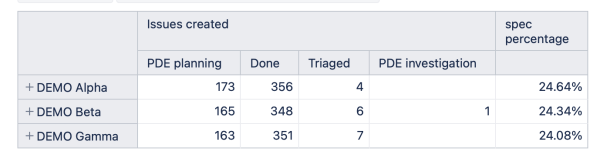Calculated Measure for Issues Created by Status

Hi,
I would like to create an additional percentage measure to this report.

New Percentage Field = (PDE Investigation + Triage)/ (PDE Investigation + Triage + Done + PDE Planning) * 100

I have the issues create broken down by status and not sure how to derive this new field.

Thanks

Hi,

I see that you have added a calculated percentage to the report - it shows the percentage of a particular row from the total of all rows.
However, if you look for a share of one or several statuses against the row total - you may use another approach.
You might create a new calculated measure that calculates the percentage of the given statuses against all statuses.
You may find out more about defining calculated measures here - Calculated measures.

Depending on whether these four statuses cover all the possibilities, you may use the first or the second option from the expression below.

--option 1 - there are multiple statuses, but only the named 4 are in the scope of report

(([Measures].[Issues created],
[Status].[PDE Planning])
+
([Measures].[Issues created],
[Status].[Triage]))
/
(([Measures].[Issues created],
[Status].[PDE Planning])
+
([Measures].[Issues created],
[Status].[Triage])
+
([Measures].[Issues created],
[Status].[Done])
+
([Measures].[Issues created],
[Status].[PDE Planning]))

--or the other option - if the 4 above options cover all the possible statuses

-- (([Measures].[Issues created],
-- [Status].[PDE Planning])
-- +
-- ([Measures].[Issues created],
-- [Status].[Triage]))
-- /
--  ([Measures].[Issues created],
--   [Status].DefaultMember)

If you want to display the result for each status for certain measures only - you may drill into the measure by the specific dimension as described here Create reports.
To do that on the already created report - please remove the Status dimension from the report columns and then perform drilling only in the measures you would like to show split.

That would lead you to a report like this.Oskars / support@eazybi.com